Home
Hostname: page-component-684899dbb8-nlvjk Total loading time: 0.338 Render date: 2022-05-22T21:27:23.270Z Has data issue: true Feature Flags: { "shouldUseShareProductTool": true, "shouldUseHypothesis": true, "isUnsiloEnabled": true, "useRatesEcommerce": false, "useNewApi": true }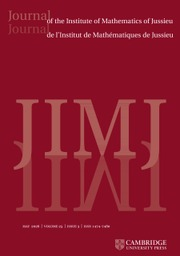Journal of the Institute of Mathematics of Jussieu

## Abstract

Let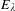$E_{\unicode[STIX]{x1D706}}$ be the Legendre family of elliptic curves. Given$n$ points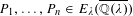$P_{1},\ldots ,P_{n}\in E_{\unicode[STIX]{x1D706}}(\overline{\mathbb{Q}(\unicode[STIX]{x1D706})})$, linearly independent over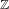$\mathbb{Z}$, we prove that there are at most finitely many complex numbers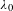$\unicode[STIX]{x1D706}_{0}$ such that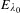$E_{\unicode[STIX]{x1D706}_{0}}$ has complex multiplication and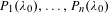$P_{1}(\unicode[STIX]{x1D706}_{0}),\ldots ,P_{n}(\unicode[STIX]{x1D706}_{0})$ are linearly dependent over End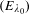$(E_{\unicode[STIX]{x1D706}_{0}})$. This implies a positive answer to a question of Bertrand and, combined with a previous work in collaboration with Capuano, proves the Zilber–Pink conjecture for a curve in a fibered power of an elliptic scheme when everything is defined over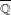$\overline{\mathbb{Q}}$.

## MSC classification

Type
Research Article
Information
Journal of the Institute of Mathematics of Jussieu , September 2019 , pp. 941 - 956

## Access options

Get access to the full version of this content by using one of the access options below. (Log in options will check for institutional or personal access. Content may require purchase if you do not have access.)

## Footnotes

F. B. was supported by the EPSRC grant EP/N007956/1’ and the SNF grant 165525.

## References

André, Y., Shimura varieties, subvarieties and CM points, Six Lectures at the Franco-Taiwan Arithmetic Festival (2001), http://math.cts.nthu.edu.tw/Mathematics/lecnotes/andre2001all.ps.Google Scholar
Barroero, F. and Capuano, L., Linear relations in families of powers of elliptic curves, Algebra Number Theory 10(1) (2016), 195214.CrossRefGoogle Scholar
Bertrand, D., Extensions de D-modules et groupes de Galois différentiels, in p-Adic Analysis (Trento, 1989), Lecture Notes in Mathematics, Volume 1454, pp. 125141 (Springer, Berlin, 1990).CrossRefGoogle Scholar
Bertrand, D., Unlikely intersections in Poincaré biextensions over elliptic schemes, Notre Dame J. Form. Log. 54(3–4) (2013), 365375.CrossRefGoogle Scholar
Bilu, Y., Masser, D. and Zannier, U., An effective ‘theorem of André’ for CM-points on a plane curve, Math. Proc. Cambridge Philos. Soc. 154(1) (2013), 145152.CrossRefGoogle Scholar
Bombieri, E. and Gubler, W., Heights in Diophantine Geometry, New Mathematical Monographs, Volume 4, (Cambridge University Press, Cambridge, 2006).Google Scholar
Breuer, F., Heights of CM points on complex affine curves, Ramanujan J. 5(3) (2001), 311317.CrossRefGoogle Scholar
Colmez, P., Sur la hauteur de Faltings des variétés abéliennes à multiplication complexe, Compos. Math. 111(3) (1998), 359368.CrossRefGoogle Scholar
David, S., Minorations de formes linéaires de logarithmes elliptiques, Mém. Soc. Math. Fr. (N.S.) 62 (1995), iv+143.Google Scholar
David, S., Points de petite hauteur sur les courbes elliptiques, J. Number Theory 64(1) (1997), 104129.CrossRefGoogle Scholar
van den Dries, L., Tame Topology and O-minimal Structures, London Mathematical Society Lecture Note Series, Volume 248 (Cambridge University Press, Cambridge, 1998).CrossRefGoogle Scholar
van den Dries, L. and Miller, C., On the real exponential field with restricted analytic functions, Israel J. Math. 85(1–3) (1994), 1956.CrossRefGoogle Scholar
van den Dries, L. and Miller, C., Geometric categories and o-minimal structures, Duke Math. J. 84(2) (1996), 497540.CrossRefGoogle Scholar
Ford, L., Automorphic Functions, second edition (American Mathematical Society, Providence, RI, 1951).Google Scholar
Galateau, A., Une minoration du minimum essentiel sur les variétés abéliennes, Comment. Math. Helv. 85(4) (2010), 775812.CrossRefGoogle Scholar
Habegger, P., Special points on fibered powers of elliptic surfaces, J. Reine Angew. Math. 685 (2013), 143179.Google Scholar
Habegger, P. and Pila, J., O-minimality and certain atypical intersections, Ann. Sci. Éc. Norm. Supér. (4) 49(4) (2016), 813858.CrossRefGoogle Scholar
Hartshorne, R., Algebraic Geometry, Graduate Texts in Mathematics, Volume 52, (Springer, New York–Heidelberg, 1977).CrossRefGoogle Scholar
Lang, S., Elliptic Functions (Addison-Wesley Publishing Co., Inc., Reading, MA–London–Amsterdam, 1973). With an appendix by J. Tate.Google Scholar
Masser, D., Linear relations on algebraic groups, in New Advances in Transcendence Theory (Durham, 1986), pp. 248262 (Cambridge University Press, Cambridge, 1988).CrossRefGoogle Scholar
Masser, D., Counting points of small height on elliptic curves, Bull. Soc. Math. France 117(2) (1989), 247265.CrossRefGoogle Scholar
Masser, D. and Zannier, U., Torsion anomalous points and families of elliptic curves, C. R. Math. Acad. Sci. Paris 346(9–10) (2008), 491494.CrossRefGoogle Scholar
Masser, D. and Zannier, U., Torsion anomalous points and families of elliptic curves, Amer. J. Math. 132(6) (2010), 16771691.Google Scholar
Masser, D. and Zannier, U., Torsion points on families of squares of elliptic curves, Math. Ann. 352(2) (2012), 453484.CrossRefGoogle Scholar
Peterzil, Y. and Starchenko, S., Uniform definability of the Weierstrass functions and generalized tori of dimension one, Selecta Math. (N.S.) 10(4) (2004), 525550.CrossRefGoogle Scholar
Pila, J., Rational points of definable sets and results of André–Oort–Manin–Mumford type, Int. Math. Res. Not. IMRN 13 (2009), 24762507.Google Scholar
Pila, J. and Zannier, U., Rational points in periodic analytic sets and the Manin–Mumford conjecture, Atti Accad. Naz. Lincei Cl. Sci. Fis. Mat. Natur. Rend. Lincei (9) Mat. Appl. 19(2) (2008), 149162.CrossRefGoogle Scholar
Pink, R., A common generalization of the conjectures of André–Oort, Manin–Mumford and Mordell–Lang. Manuscript dated 17th April, 2005.Google Scholar
Silverman, J. H., Heights and the specialization map for families of abelian varieties, J. Reine Angew. Math. 342 (1983), 197211.Google Scholar
Silverman, J. H., The Arithmetic of Elliptic Curves, second edition, Graduate Texts in Mathematics, Volume 106, (Springer, Dordrecht, 2009).CrossRefGoogle Scholar
Viada, E., The intersection of a curve with a union of translated codimension-two subgroups in a power of an elliptic curve, Algebra Number Theory 2(3) (2008), 249298.CrossRefGoogle Scholar
Zannier, U., Some Problems of Unlikely Intersections in Arithmetic and Geometry, Annals of Mathematics Studies, Volume 181, (Princeton University Press, 2012). With appendixes by David Masser.Google Scholar
Zimmer, H. G., On the difference of the Weil height and the Néron–Tate height, Math. Z. 147(1) (1976), 3551.CrossRefGoogle Scholar

1
Cited by

# Save article to Kindle

Note you can select to save to either the @free.kindle.com or @kindle.com variations. ‘@free.kindle.com’ emails are free but can only be saved to your device when it is connected to wi-fi. ‘@kindle.com’ emails can be delivered even when you are not connected to wi-fi, but note that service fees apply.

Find out more about the Kindle Personal Document Service.

CM RELATIONS IN FIBERED POWERS OF ELLIPTIC FAMILIES
Available formats
×

# Save article to Dropbox

To save this article to your Dropbox account, please select one or more formats and confirm that you agree to abide by our usage policies. If this is the first time you used this feature, you will be asked to authorise Cambridge Core to connect with your Dropbox account. Find out more about saving content to Dropbox.

CM RELATIONS IN FIBERED POWERS OF ELLIPTIC FAMILIES
Available formats
×

# Save article to Google Drive

To save this article to your Google Drive account, please select one or more formats and confirm that you agree to abide by our usage policies. If this is the first time you used this feature, you will be asked to authorise Cambridge Core to connect with your Google Drive account. Find out more about saving content to Google Drive.

CM RELATIONS IN FIBERED POWERS OF ELLIPTIC FAMILIES
Available formats
×
×